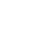# Fine-Tuning Myel Network EconomicsA. CamutoA. Camuto

Modelling the economics of the Myel network is not trivial, but we've created some simple statistical models to help us inform some of our pricing choices as we roll out our network.

The Myel network is a carefully calibrated balancing act between offering sufficient incentives for peers to participate and remain online, but also keeping costs low across the network to keep applications and developers satisfied.

### The Network Actors

In the first model of this series we focus on a single application deployed on the Myel network that has $N$ users, with an average payload of $B$ bytes. We assume that the application has a proportion $d\in[0,1]$ of users that recur daily. On a given day we thus have:

$\mathrm{daily\_users} = d\times N$ users.

Let's assume that these users load the app daily according to a Poisson distribution, i.e most users load the app a few times, but a couple really love it and use it often.

$\mathrm{daily\_uses} \sim \mathrm{Poisson(\lambda)}$, where $\lambda$ controls how often the average user loads the app.

Now we assume that a proportion $p$ of those $N$ users are also providers of the application's content. They serve the content to all the other users. Here we assume that because the Myel plugin is a background process that runs continuously on someone's computer that the providers have a probability of being online on a given day $m\in[0,1]$, that is distinct from $d$ and often larger than $d$ such that we have $m\geq d$. Basically we are assuming that an application's providers are often more likely to be present on a given day than an application's users. On a given day we thus have:

$\mathrm{daily\_providers} = m\times p \times N$ providers.

Now some of those providers might also be users on a given day ! Assuming that providers are also likely to use an app with a probability $d$ on a given day, we have a special class of provider that already has the content they need to use the app ! On a given day we have:

$\mathrm{daily\_providers} \ \cap \mathrm{daily\_users} = d \times p \times N$ providers that are also using the app.

### Content Transmission

We've now established the different types of actors on the Myel network. We now need to make assumptions as to how content is transmitted. We assume that we have a population of users that have locations that are distributed equally across a square area.

$\mathrm{location} \sim \mathrm{Uniform(\mathrm{square})}$.

We now assume that application users get their content from the nearest provider. The uniform assumption means that on average each provider serves daily:

$\mathrm{daily\_users\_per\_provider}=\frac{\mathrm{daily\_users} - \mathrm{daily\_providers} \ \cap \mathrm{daily\_users}}{\mathrm{daily\_providers}}=\frac{d(1-p)}{m\times p}$

Lets say the payload of the app is $M$ bytes, and we have a $\mathrm{price}$ per byte delivered (in FIL or USD).

Each provider earns on average per day:

$\mathrm{daily\_provider\_earnings} = \mathrm{price}\times \mathrm{users\_per\_provider\_daily} \times \mathbb{E}(\mathrm{daily\_uses}) = \mathrm{price} \times M \times \frac{d(1- p)}{m\times p} \times \lambda$

This comes from the fact that the expected value $\mathbb{E}$ of a Poisson distribution is just its characteristic parameter $\lambda$.

And the network generates a total earnings of

$\mathrm{daily\_total\_earnings} = \mathrm{daily\_providers} \times \mathrm{price}\times \mathrm{users\_per\_provider\_daily} \times \mathbb{E}(\mathrm{daily\_uses}) = N \times \mathrm{price} \times M \times d(1-p)\times \lambda$

### Balancing Earnings and Network Performance

There’s a number of factors here we can’t control as the designers of the network. We can’t control the number of users $N$, the proportion of daily users $d$, the size of the application content $M$, the proportion of providers online on a given day $m$, or the average uses per user $\lambda$. But we can act on the $\mathrm{price}$ and control the proportion of users that are providers $p$.

As $p$ increases we can assume that performance of the network increases, there are more providers to serve content quickly to users, but the returns per provider and for the network as a whole decrease ! Thus, given a fixed agreed upon $\mathrm{price}$, controlling $p$ is where we can have the most impact -- carefully balancing and fine-tuning network performance against strong earnings for both the network and for individual providers. As a concrete example consider the case where all users are providers, $p=1$. Because all users already have the content, there are no bytes to be delivered, and no one earns anything !

This kind of modelling will be critical as we roll out the network and need to make informed decisions on controlling $p$. We’ll start shipping more sophisticated models where we assume more complex location distributions, and as we gather data on the network we can also model network performance as a function of $p$.

### Simulations

Consider an application with 2000 users ($N=2000$) of which $40\%$ use the app on a given day ($d=0.4$), content delivery is priced at 0.08USD/GiB, the application content size is $M=$0.1GiB, the provider uptime is roughly $m=0.7$, the proportion of providers is $p=0.01$, and finally most users use the app twice a day $\lambda=2$.

Providers earn roughly 304USD a year and the network yield roughly 4204.8USD.

Below we show a simulation for these values, but where $N=200$ and $p=0.1$ - so as to not clutter the network visualisation. Black Nodes represent network providers, red nodes are peers that are active on a given day, and grey nodes are offline peers